# Math Challenge - July 2019

• Challenge
• Featured
Mentor
Questions

1.
(solved by @Flatlanderr , solved by @lriuui0x0 ) Show that ##\frac{\pi}{4} + \frac{3}{25} \lt \arctan \frac{4}{3} \lt \frac{\pi}{4} + \frac{1}{6}##

2. (solved by @nuuskur ) Show that the equation ##x + x^3 + x^5 + x^7 = {c_1}^2 (c_1 - x) + {c_2}^2 (c_2 - x)## where ##c_1, c_2 \in \mathbb{R}##, has only one real root.

3. (solved by @KnotTheorist ) Let ##A = \begin{bmatrix}
7 & 3\\
3 & -1
\end{bmatrix}##. Find an orthogonal matrix ##P## such that ##D = P^{-1} A P## is diagonal.

4. (solved by @nuuskur ) Using Calculus, show that the roots of the equation ##x^5 + ax^4 + bx^3 + cx^2 + dx + e = 0## cannot be all real if ##2a^2 \lt 5b##

5. Consider the projective space ##P^m(F_q) = P(F_q^{m+1})##. How many (t+1)-dimensional spaces in this projective space contain a given t-dimensional space?

6. (solved by @nuuskur ) Let ##C## be a subspace of ##F_q^n##. We call ##C## cyclic if ##C## contains its right shifts, i.e. ##x = (c_1, \dots, c_n) \in C \implies \vec{x} = (c_n, c_1 \dots, c_{n-1}) \in C##. Show that there is a correspondence between the cyclic subspaces of ##F_q^n## and the ideals of the quotient ring ##F_q[X]/(X^n-1)##.

7. (solved by @nuuskur ) Let ##(W_t)_{t\geq 0}## be a Brownian motion on a suitable probability space. Put ##X:= \int_{0}^t W_s^2 ds##. Calculate ##E(X).## (changed, 7/5/19)

8. (solved by @nuuskur ) Calculate the Galois group of the splitting field of ##x^4 - 2x^2 -2## over ##\mathbb{Q}##. Determine the isomorph type of the Galois group.

9. Prove that every symmetric and positive definite matrix ##A\in \mathbb{M}(n,\mathbb{R})## can be uniquely written as ##A=L\cdot L^\tau##, where ##L## is a lower triangular matrix with positive diagonal elements.
Calculate ##L## for ##A=\begin{bmatrix}4 &2& 4& 4\\
2& 10& 17& 11\\
4& 17& 33& 29\\
4& 11& 29& 39\end{bmatrix}##.

10. (solved by @nuuskur ) Let ##L\subseteq H## be a nonempty, closed, and convex set in a Hilbert space. Prove that there is an element of minimal norm in ##L##.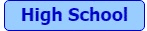11. (solved by @Physics lover ) Solve the following integral
$$I = \int_0^{\pi/2} \frac{\sin^{2019} x}{\cos^{2019} x + \sin^{2019} x}dx$$

12. (solved by @Pi-is-3 ) Is ##N:=21^{39}+39^{21}## divisible by ##45##? Why, why not?

13. (solved by @Pi-is-3 ) Let ##0<u,v,w<1##. Show that among the numbers ##u(1-v)\, , \,v(1-w)\, , \,w(1-u)## is at least one value not greater than ##\dfrac{1}{4}\,.##

14. (solved by @JosephFG ) What is the ratio between the red and the blue area where ##P## and ##Q## are one fifth away from the vertices?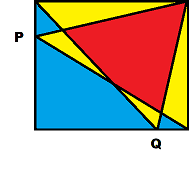15. (solved by @Pi-is-3 ) In what ratio does the circumference of the circle divide the left and right sides of the square?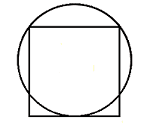Last edited:
•Pi-is-3, YoungPhysicist, member 587159 and 1 other person

My Solution to 3
This is just a simple eigenvalue problem, where P is just the matrix of eigenvectors, which if the eigenvectors themselves are normalized, will become an orthogonal matrix. And then it will diagonalize to its eigenvalues.
We can get that the eigenvalues of A are 8 and -2, then solving for the eigenvectors, ensuring that they are normalized, we get v1 = 1/√10 (3 1) and v2 = 1/√10 (-1 3).
P =
\begin{pmatrix}
3/√10 & -1/√10 \\
1/√10 & 3/√10
\end{pmatrix}

And then P-1 = PT. Then solving for D, we get D = P-1 A P = PT A P =
\begin{pmatrix}
8 & 0 \\
0 & -2
\end{pmatrix}

•QuantumQuest
Question 1:

##\frac{\pi}{4} = \arctan 1##

Therefore we get ##\frac{3}{25} < \arctan \frac{4}{3} -\arctan 1 < \frac{1}{6}##.

Since

\begin{aligned} \tan (a - b) &= \frac{\tan a - \tan b}{1 + \tan a \tan b} \\ a - b &= \arctan \frac{\tan a - \tan b}{1 + \tan a \tan b} \end{aligned}

Let ##a = \arctan x## and ##b = \arctan y##,

we have

$$\arctan x - \arctan y = \arctan \frac{a - b}{1 + ab}$$

Therefore we need to prove ##\frac{3}{25} < \arctan \frac{1}{7} < \frac{1}{6}##.

Expand ##\arctan x## with power series, we have ##\arctan x = \sum (-1)^k \frac{x^{2k + 1}}{2k + 1}\; (k \ge 0)##. Denote partial sum as ##S_k##, since the power series is an alternating series whose absolute term value is monotonically decreasing, we can prove the greatest partial sum is ##S_0##, the lowest partial sum is ##S_1##. Therefore ##x - \frac{x^3}{3} < \arctan x < x##. Put in value ##\frac{1}{7}##, we get the inequality.

To prove ##S_0## and ##S_1## are maximum and minimum partial sum respectively, first note that ##S_{2k} > S_{2k + 1}##, then note ##S_{2k} > S_{2k + 2}## and ##S_{2k + 1} < S_{2k + 3}##.

QuantumQuest
Gold Member
Let ##a = \arctan x## and ##b = \arctan y##,

we have

## \arctan x - \arctan y = \arctan \frac{a - b}{1 + ab}##

How did you get this?

Hi QuantumQuest,

So given ##a=\arctan x## and ##b=\arctan y##, put ##a## and ##b## into previous equation, we get

\begin{aligned} a − b &= \arctan \frac{\tan a − \tan b}{1+ \tan a \tan b} \\ \arctan x - \arctan y &= \arctan \frac{\tan \arctan x - \tan \arctan y}{1 + (\tan \arctan x)(\tan \arctan y)} \\ \arctan x - \arctan y &= \arctan \frac{x - y}{1 + xy} \end{aligned}

•QuantumQuest
Some ideas for the second problem.
Replace $c_1 =: u, c_2 =: v$. Assume there is a (real) root $r$ for the polynomial. Transform initial problem to comparison of coefficients as follows.
\begin{align*} &x^7 + x^5 + x^3 + (1+u^2 + v^2)x - u^3 - v^3 \\ = &(x-r)(a_6x^6 + a_5x^5 + a_4x^4 + a_3x^3 + a_2x^2 + a_1x + a_0) \end{align*}
It is now a matter of finding what $r$ is in terms of the parameters $u,v$. If there is no branching, then $r$ is necessarely unique.

The coefficients are restricted as follows.
\begin{align*} &a_6 = 1 \\ &a_5 = a_6r &a_4 = a_5r + 1 \\ &a_3 = a_4r &a_2 = a_3r + 1 \\ &a_1 = a_2r &a_0 - a_1r = 1+u^2 + v^2 \\ &a_0r = u^3 + v^3 \end{align*}
Using
$$\begin{cases}a_0r = u^3 + v^3 \\ a_0 - a_1r = 1+ u^2+v^2 \end{cases}$$
multiplying both sides of the second equality by $r$ we get
$$a_0r - a_1r^2 = (1+u^2+v^2)r \iff a_1r^2 + (1+u^2+v^2)r - (u^3+v^3) = 0.$$
For $r$ to be unique, we require the discriminant
$$(1+u^2+v^2)^2 + 4a_1(u^3+v^3) = 0.$$
By tracing the restrictions set for the coefficients $a_0,\ldots , a_6$, we arrive at
$$a_1 = r^5 + r^3 + r$$
I predict some sort of guesswork ahead..
Not enough brainpower left at the moment. To be continued..

For problem 2
The equation can be rearranged to be-
$$f(x)= x^7 + x^5 + x^3 + (c_1^2+c_2^2)x-(c_1^3+c_2^3)=0$$
By Descartes rule of signs, this problem is trivialized.

Let ( c_1^2+c_2^2)=k, k \ge 0 Let (c_1^3+c_2^3)=t [\tex] If t>0, f(x) has exactly one real root, by Descartes rool of signs. If t<0, then f(-x) has exactly one real root, which is the same thing. https://en.wikipedia.org/wiki/Descartes'_rule_of_signs Can I also contribute to maths challenge from next time? Question 12 is trivial N:=213 21^{39}+39^{21} (mod 9)=0 21^{39}+39^{21} (mod 5)=1-1=0 Hence it is divisible by 45 EDIT: Alternate 21^39 is divisible by 9 because it has 3 raised to a power more than 2. Similarly with 39^21. Binomial expansion of (20+1)^39= 20^39+ (39)(20^38)+...+(39)(20)+1 which leaves 1 as remainder with five. (40-1)^21 leaves 4 remainder on the other hand. Adding them up leaves no remainder. Hence, the sum is divisible by 45. @fresh_42 New proof added. I am in highschool BTW (11th grade). Last edited: Mentor Can I also contribute to maths challenge from next time? If you like send me a PM, if possible with better LaTeX ;-) Mentor Question 12 is trivial N:=213 21^{39}+39^{21} (mod 9)=0 21^{39}+39^{21} (mod 5)=1-1=0 Hence it is divisible by 45 We need a proof, not a computer calculation. •Auto-Didact We need a proof, not a computer calculation. I didn't do a computer calculation. It's simple modular arithmetic. a^n (mod k)= (a (mod k))^n for a being integer, and n and k being a natural numbers. https://brilliant.org/wiki/modular-arithmetic/ Mentor I didn't do a computer calculation. It's simple modular arithmetic. a^n (mod k)= (a (mod k))^n for a being integer, and n and k being a natural numbers. https://brilliant.org/wiki/modular-arithmetic/ Can you write this in a form that high schoolers can read and understand it? You do not have to convince me! •Pi-is-3 QuantumQuest Science Advisor Gold Member So given ... \begin{aligned} a − b &= \arctan \frac{\tan a − \tan b}{1+ \tan a \tan b} \\ \arctan x - \arctan y &= \arctan \frac{\tan \arctan x - \tan \arctan y}{1 + (\tan \arctan x)(\tan \arctan y)} \\ \arctan x - \arctan y &= \arctan \frac{x - y}{1 + xy} \end{aligned} Yes, last line is different from what you wrote in post #3 and it is correct now. With this corrected your solution is correct. Well done. QuantumQuest Science Advisor Gold Member Regarding Question ##1## there is a simpler solution using just Calculus I. So, anyone other than lriuui0x0 - who has already got the credit, can also take credit if he / she finds it. QuantumQuest Science Advisor Gold Member For problem 2 Sorry for bad latex. You're missing an ##x## in your ##f(x)##. Also, please write in Latex - you can take a look at "INFO"##\rightarrow## "Help"##\rightarrow## "LaTeX Primer" (top navigation bar) if you don't know about Latex. Moreover, the problem states that ##c_1, c_2 \in \mathbb{R}##. What if they are both zero? Last edited: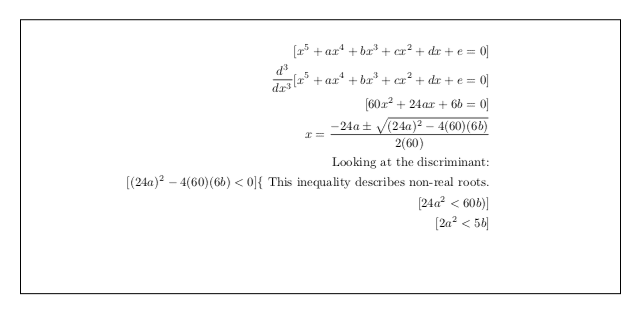Question 4 :) QuantumQuest Science Advisor Gold Member Question 4 :) You take the third derivative as I see. What theorem are you using here? How do you get to the last two lines? Also, why is the answer in the form of an image? I may have overthought about problem 2. Fix $u,v\in\mathbb R$ and set [tex] f(x) = x^7 + x^5 + x^3 + (1+u^2+v^2)x - (u^3+v^3).
Its derivative is
$$f'(x) = 7x^6 + 5x^4 + 3x^2 + 1+u^2+v^2.$$
Since $f$ is of odd degree it necessarily has at least one real root by the intermediate value theorem. It also holds that $f'(x) >0$ for every $x\in\mathbb R$. Thus $f$ is strictly increasing, hence there can only be one real root.
I think my previous idea will fail, because the solution would be given implicitly - thus there being no progress.
Proof by contrapositive. Assume the polynomial has all real roots and set
$$f(x) = (x-a_5)(x-a_4)(x-a_3)(x-a_2)(x-a_1)$$
We are interested in the coefficients of $x^3, x^4$, we get
$$a = -(a_1+a_2+a_3+a_4+a_5) \\ b = a_1a_2 + a_1a_3 + a_1a_4 + a_1a_5 + a_2a_3 + a_2a_4 + a_2a_5 + a_3a_4 + a_3a_5 + a_4a_5$$
Study relationship between quantities $2a^2$ and $5b$. Post expansion we have
$$a^2 = a_1^2 + a_2^2 + a_3^2 + a_4^2 + a_5^2 + \\ 2 \lbrace a_1a_2 + a_1a_3 + a_1a_4 + a_1a_5 + a_2a_3 + a_2a_4 + a_2a_5 + a_3a_4 + a_3a_5 + a_4a_5 \rbrace =: c + 2b.$$
Finally we have to show $2a^2 \geq 5b$ i.e $2(c+2b) \geq 5b$ i.e $2c \geq b$. This is somewhat immediate, if a bit technical. We show $4c \geq 2b$. Group as follows to the left side
$$(a_1^2 - 2a_1a_2 + a_2^2) + (a_1^2 - 2a_1a_3 + a_3^2) + (a_1^2 - 2a_1a_4 + a_4^2) + (a_1^2 - 2a_1a_5 + a_5^2)$$
with this we have exhausted four copies of $a_1^2$ and the quantity above is non-negative. Proceed with
$$(a_2^2 - 2a_2a_3 + a_3^2) + (a_2^2 - 2a_2a_4 + a_4^2) + (a_2^2 - 2a_2a_5 + a_5^2)$$
now all copies of $a_2^2$ are exhausted and the quantity remains non-negative. Proceed similarly and obtain $4c - 2b\geq 0$. This concludes the proof.
I noticed that P4 required the use of calculus?What, precisely, does that mean? If my approach doesn't qualify, it's fine.
Again, proof by contrapositive. We could make use of the Gauss-Lucas theorem, which states that the zeros of $f'$ are convex combinations of the zeros of $f$. If we assumed all the roots of $f$ are real, by Gauss Lucas, all the roots of derivatives of arbitrary order are also real. Thus, taking the third derivative
$$f^{(3)}(x) = 60x^2 + 24ax + 6b$$
and because the roots are real, we have the following restriction:
$$(24a)^2 - 1440b \geq 0 \iff 2a^2 \geq 5b.$$

Last edited:
•QuantumQuest
If I understand this correctly, we need to show that $L$ contains an element $u$ which satisfies
$$\|u\| = \inf _{x\in L} \|x\|$$
Making use of the parallelogram law we get for any $x,a,b\in H$
$$\|(x-a) + (x-b)\|^2 + \|(x-a)-(x-b)\|^2 = 2(\|x-a\|^2 + \|x-b\|^2)$$
Calculating the lhs of the above and by re-arranging we get to
$$\|a-b\|^2 = 2\|x-a\|^2 + 2\|x-b\|^2 - 4\|x- \frac{1}{2}(a+b)\|^2\tag{E}$$
In light of P10, we can take $x=0$, but we can solve this problem more generally, fix $x\in H$. Set $l := \inf _{y\in L} \|x-y\|$. If we take $a,b\in L$ then due to convexity $\frac{1}{2}(a+b) \in L$, therefore
$$l \leq \|x-\frac{1}{2}(a+b)\|.$$
Per definition of infimum, there exist sequences that converge to it, so pick $a_n\in L, n\in\mathbb N$ such that
$$\|x-a_n\|^2 < l^2 + \frac{1}{n}$$
By (E) we then have for all $m,n\in \mathbb N$
$$\|a_n-a_m\| \leq 2\|x-a_n\|^2 + 2\|x-a_m\|^2 -4l^2 \\ \leq 2l^2 + \frac{2}{n} + 2l^2 + \frac{2}{m} - 4l^2 = \frac{2}{n} + \frac{2}{m}$$
This mean the sequence $(a_n)$ is Cauchy and by completeness $a_n \xrightarrow[]{}u \in H$. Due to closedness $u\in L$. Consequently
$$\|x-u\|^2 = \lim _{n\to\infty} \|x-a_n\|^2 = l^2.$$
In fact, this $u$ is unique, for if $v$ satisfies the same demand, then by (E)
$$\|u-v\| \leq 2\|x-u\|^2 + 2\|x-v\|^2 -4l^2 = 2l^2 + 2l^2 - 4l^2 = 0.$$
Geometrically, this makes sense. For instance, in case of a ball on a plane, for a fixed point in the space, one can always pick a point on the ball closest to that fixed point.
If the fixed point is contained in the ball, the closest point to it is itself, of course. If we give up convexity, the closest point need not be unique. For instance, unit sphere is not convex, there are infinitely many points at distance $1$ from the origin.

Last edited:
•fresh_42
Regarding Question ##1## there is a simpler solution using just Calculus I. So, anyone other than lriuui0x0 - who has already got the credit, can also take credit if he / she finds it.

Let ##f(x) = arctan(x).##

Since ##arctan(x)## is differentiable for all x, by the Mean Value Theorem we know
$$\frac{f(b) - f(a)}{b - a} = f'(c)$$
for some c on the interval ##a < c < b##.

##f'(x) = \frac{1}{1 + x^2}##, which is decreasing for ##x > 0##, so for ##0 < a < c < b##, ##f'(b) < f'(c) < f'(a)##.

Substituting, we have
$$f'(b) < \frac{f(b) - f(a)}{b - a} < f'(a)$$
$$\frac{1}{1 + b^2} < \frac{arctan(b) - arctan(a)}{b - a} < \frac{1}{1 + a^2}$$
$$\frac{b - a}{1 + b^2} < arctan(b) - arctan(a) < \frac{b - a}{1 + a^2}$$

Let ##a = 1, b = 4/3##.

$$\frac{\frac{4}{3} - 1}{1 + (\frac{4}{3})^2} < arctan(4/3) - arctan(1) < \frac{\frac{4}{3} - 1}{1 + 1^2}$$
$$\frac{3}{25} < arctan(4/3) - \frac{\pi}{4} < 1/6$$
$$\frac{\pi}{4} + \frac{3}{25} < arctan(4/3) < \frac{\pi}{4} + \frac{1}{6}$$

•mfb, QuantumQuest and fresh_42
My solution to 11.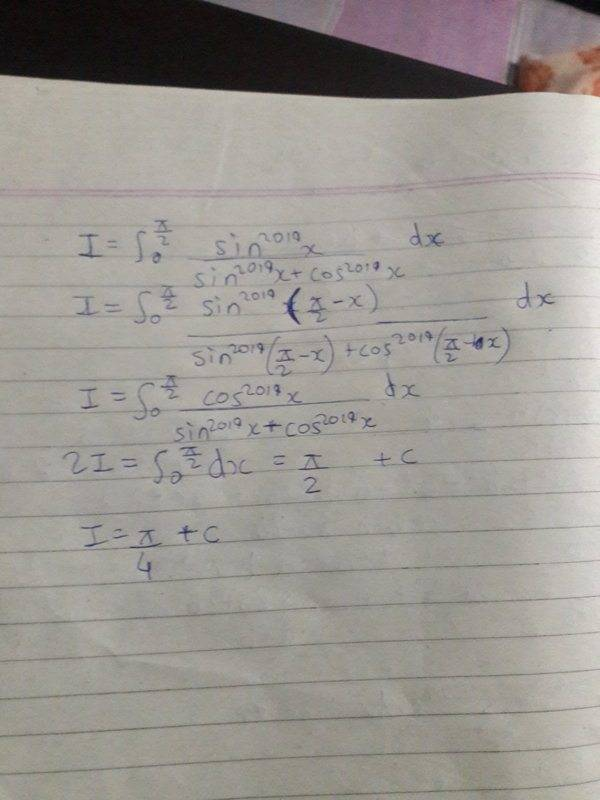By the way i wanted to know whether can we solve the same integral but indefinite.If we can please provide a solution.

member 587159
My solution to 11.
View attachment 246120

By the way i wanted to know whether can we solve the same integral but indefinite.If we can please provide a solution.

Why is there +c? It is a definite integral! For the rest,you are correct. I doubt you can find an elementary primitive. This was the solution I had in mind.

•fresh_42
Why is there +c? It is a definite integral! For the rest,you are correct. I doubt you can find an elementary primitive. This was the solution I had in mind.
Oh sorry for that c.Ok i will try for another method Can we solve its indefinite one?

Mentor
•member 587159